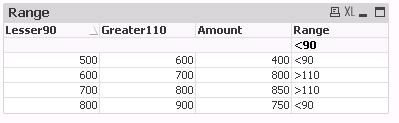New to QlikView

Discussion board where members can get started with QlikView.

Highlighted
Contributor

kindly help out to write the expression on the below condition

HI All,

i am having the column as  below ,

 Lesser 90 Greater 110 Amount 500 600 400 600 700 800 700 800 850 800 900 750

i need to write the expression

if the amount is fall under lesser 90 it should display <90%

if the amount is fall under Greater 110 it should display <110

Below is the expected Result:

 Lesser 90 Greater 110 Amount Variation 500 600 400 <90% 600 700 800 >110% 700 800 850 >110 800 900 750 <90%

Please help me out how to write the expression for this ,Very urgent requirement !.

10 Replies
Valued Contributor II

Re: kindly help out to write the expression on the below condition

Try

=if([Amount] >= [Lesser 90],

if([Amount] <= [Greater 110], 'In Range', '>100%'), '<90%')

Esteemed Contributor III

Re: kindly help out to write the expression on the below condition

Another way of doing this like

Comparison:
LOAD *, (Greater110 - Lesser90) + Amount AS TotalDiffAmt INLINE [
Lesser90, Greater110, Amount
500, 600, 400
600, 700, 800
700, 800, 850
800, 900, 750
]
;

Use straight table with

Dim: Lesser90, Greate90, Amount

Expr: = IF(TotalDiffAmt >= Greater110, '>110', '<90')Contributor

Re: kindly help out to write the expression on the below condition

i have used the below expression

=if([Amount]<= [Lesser 90],

if([Amount] >= [Greater 110], 'In Range', '>100%'), '<90%')

it is displaying value as <90 and >100% percent for all the value,

for example

lesser 90 Greater 110 amount

400 450 350

from above the amount is not present in Amount value ,and it should display 'N/A'

but it is displaying <90%

Kindly help me out

Contributor

Re: kindly help out to write the expression on the below condition

it is the Fact Table and i dont need to disturb that table,please kindly let me know the alternative solutionPartner

Re: kindly help out to write the expression on the below condition

Hi

Try the following

=IF([Amount] < [Lesser 90], '<90', IF([Amount] <= [Greater 110], '<110', '>110'))MVP

Re: kindly help out to write the expression on the below condition

Like this then:

=If(Len(TotalDiffAmt) = 0, 'N/A', If(TotalDiffAmt >= [Greater 110], '>110%', '<90%'))

Or

If(Len(TotalDiffAmt) = 0, 'N/A',

If(TotalDiffAmt > [Greater 110], '>110%',

If(TotalDiffAmt < [Lesser 90], '<90%',

'90-110'))

)

Logic will get you from a to b. Imagination will take you everywhere. - A EinsteinMVP

Re: kindly help out to write the expression on the below condition

Will you provide sample application to test? Will implement without Touch the script. If not, We can do within script

Before develop something, think If placed (The Right information | To the right people | At the Right time | In the Right place | With the Right context)
Honored Contributor

Re: kindly help out to write the expression on the below condition

Try in script

T:LOAD * INLINE [

Lesser 90, Greater 110, Amount

500, 600, 400

600, 700, 800

700, 800, 850

800, 900, 750

];

F:

load  [Lesser 90], [Greater 110], Amount,

if (Amount >[Greater 110],'>110%',if(Amount<[Lesser 90],'<90%')) as Variance

Resident T;

drop table TPartner

Re: kindly help out to write the expression on the below condition

Hi,

You're not quite precise in your requirements... Looking into initial thread- there should be only <90% and >110% result values.

Then looking into discussion, as far as I understood there should be 4 different statements:

'<90%' if amount<[lesser 90]

'In Range' if (amount >=[lesser 90] and amount <= [greater 110])

'>110%' if (amount > [greater 110])

'N/A' if (amount is null or empty or not a number)

Assuming above try the following:

if(amount < [lesser 90], '<90%',

if(amount < [greater 110], 'In Range',

if(amount  > [greater 110], '>110%', 'N/A' )

)

)

Hope this helps.

//Andrei